# Dilaton quantum gravity and cosmology Dilaton quantum gravity

• Slides: 60Dilaton quantum gravity and cosmologyDilaton quantum gravity Functional renormalization flow, with truncation :Functional renormalization equations Percacci,Fixed point for large scalar field This fixed point describes already realistic gravity !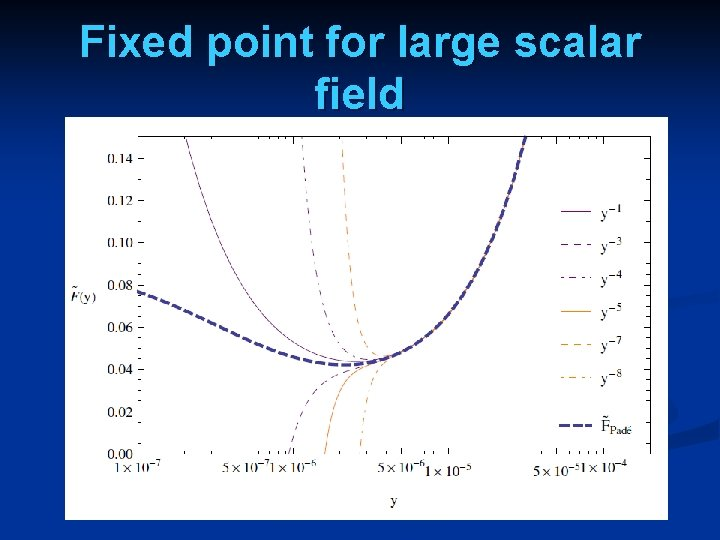Fixed point for large scalar field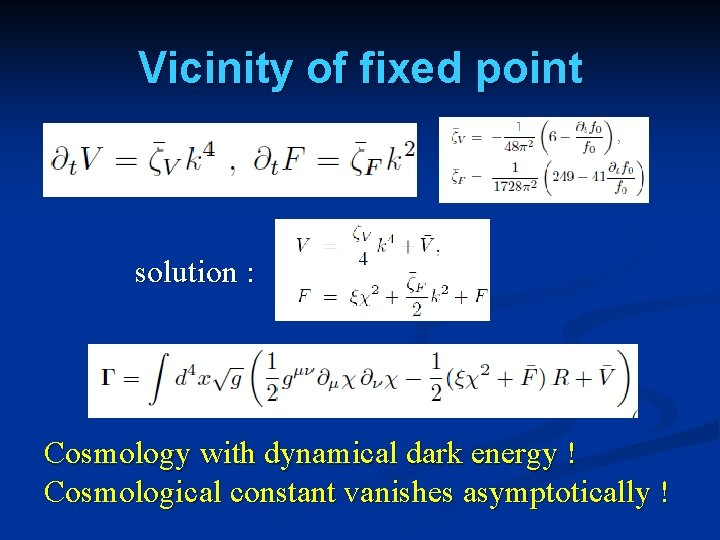Vicinity of fixed point solution : Cosmology with dynamical dark energy ! Cosmological constant vanishes asymptotically !open … full scaling solution n behavior for negative kinetic term close to conformal value na guess for dilaton quantum gravity and its cosmological consequence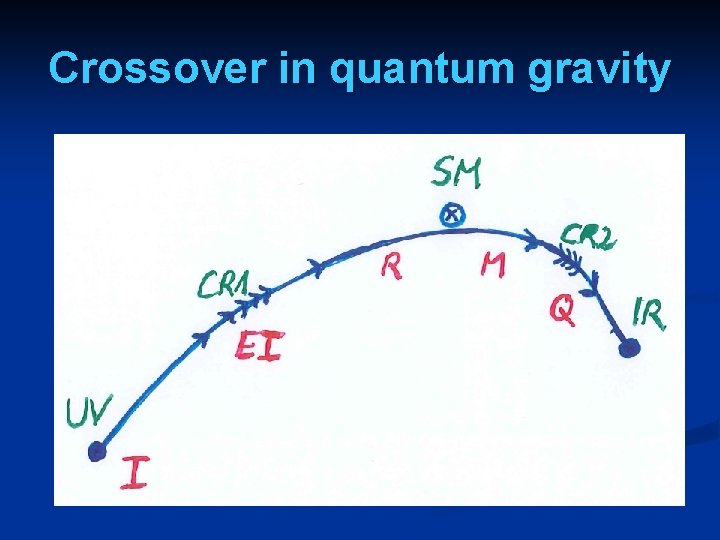Crossover in quantum gravity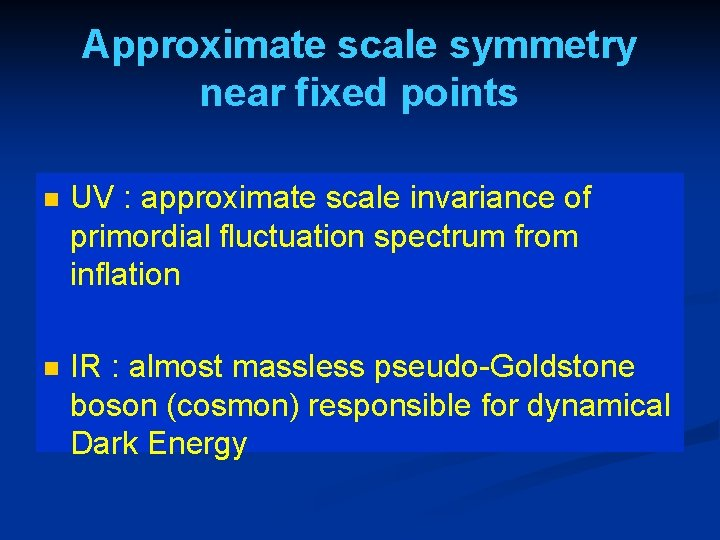Approximate scale symmetry near fixed points n UV : approximate scale invariance of primordial fluctuation spectrum from inflation n IR : almost massless pseudo-Goldstone boson (cosmon) responsible for dynamical Dark Energy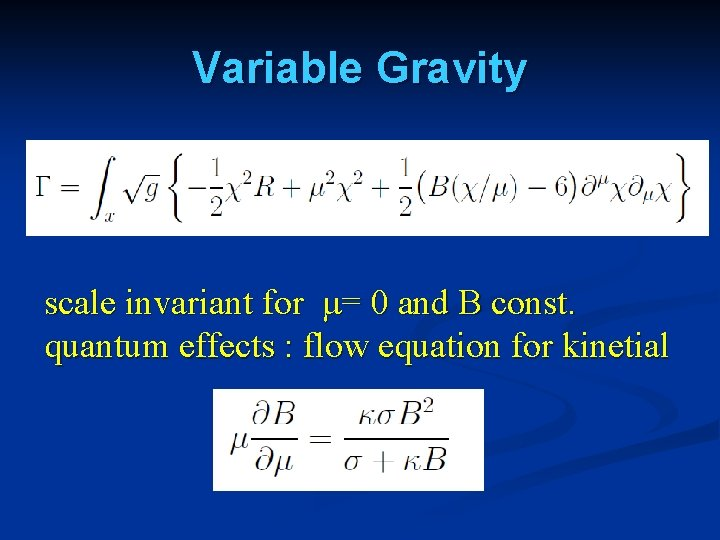Variable Gravity scale invariant for μ= 0 and B const. quantum effects : flow equation for kinetial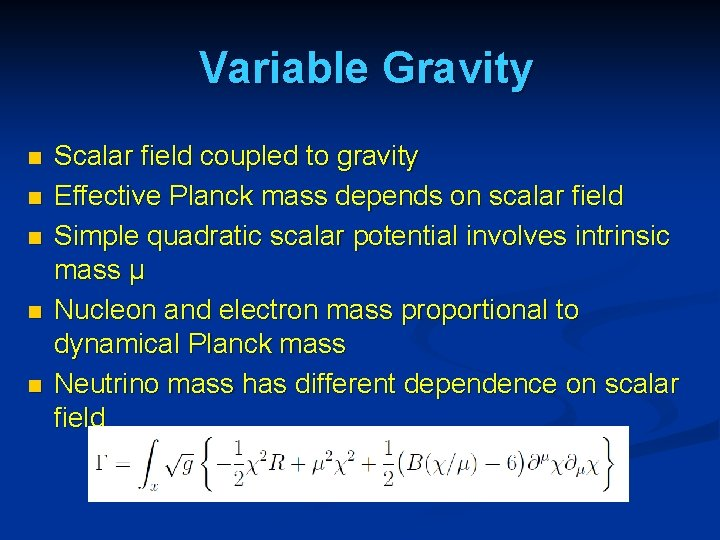Variable Gravity n n n Scalar field coupled to gravity Effective Planck mass depends on scalar field Simple quadratic scalar potential involves intrinsic mass μ Nucleon and electron mass proportional to dynamical Planck mass Neutrino mass has different dependence on scalar field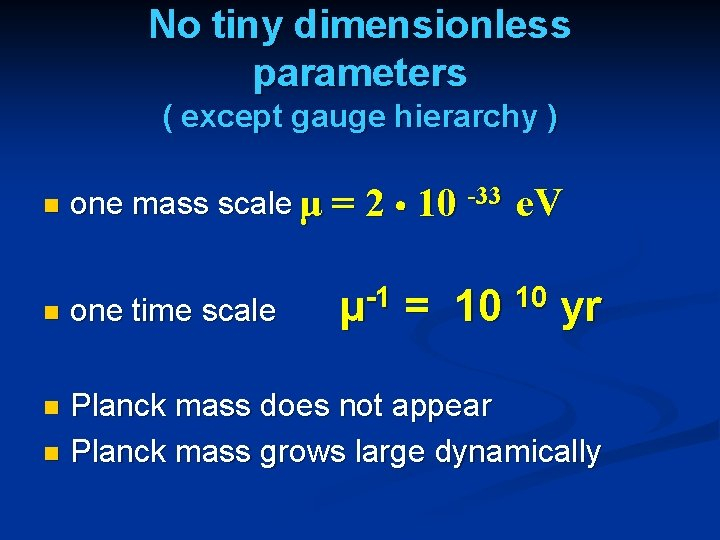No tiny dimensionless parameters ( except gauge hierarchy ) n n one mass scale μ = 2 10 -33 one time scale -1 μ = 10 e. V 10 yr Planck mass does not appear n Planck mass grows large dynamically nInfrared fixed point n μ→ 0 n B→ 0 n no intrinsic mass scale n scale symmetryFixed points and limits for scalar field n n n Dimensionless functions as B depend only on ratio μ/χ. IR: μ→ 0 , χ→∞ / y →∞ UV: μ→∞ , χ→ 0 / y → 0 Cosmology makes crossover between fixed points by variation of χ.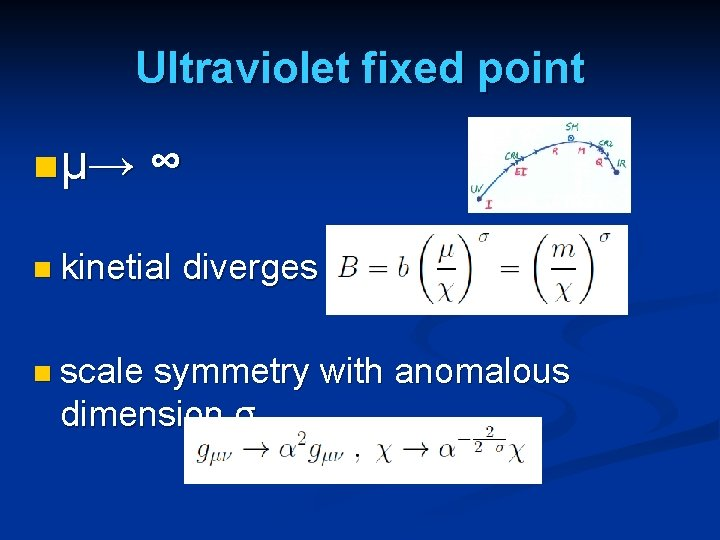Ultraviolet fixed point n μ→ ∞ n kinetial n scale diverges symmetry with anomalous dimension σ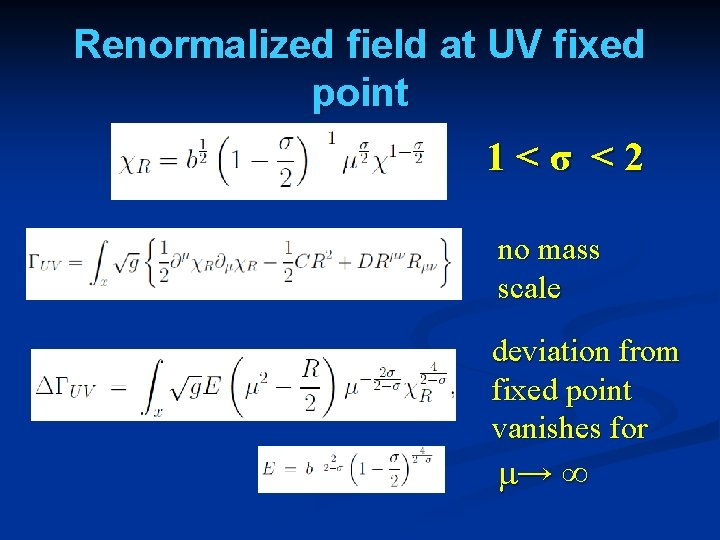Renormalized field at UV fixed point 1<σ <2 no mass scale deviation from fixed point vanishes for μ→ ∞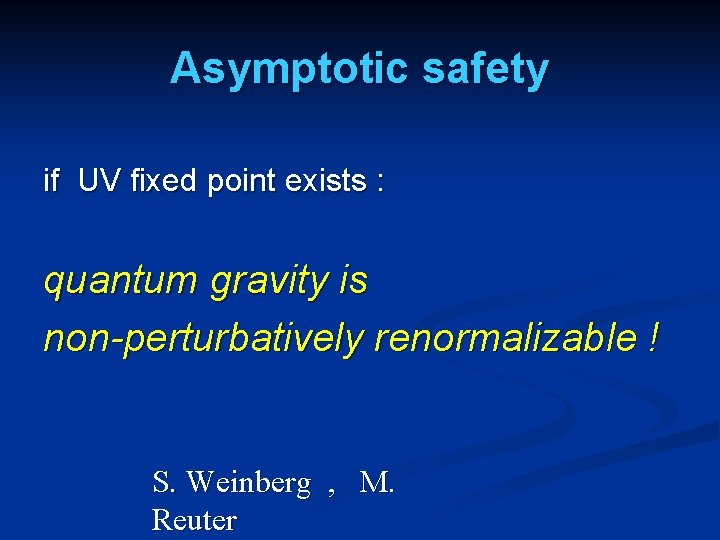Asymptotic safety if UV fixed point exists : quantum gravity is non-perturbatively renormalizable ! S. Weinberg , M. ReuterQuantum scale symmetry quantum fluctuations violate scale symmetry n running dimensionless couplings n at fixed points , scale symmetry is exact ! nCrossover between two fixed points m : scale of crossover can be exponentially larger than intrinsic scale μOrigin of mass n UV fixed point : scale symmetry unbroken all particles are massless n IR fixed point : scale symmetry spontaneously broken, massive particles , massless dilaton n crossover : explicit mass scale μ or m important n SM fixed point : approximate scale symmetry spontaneously broken, massive particles , almost massless cosmon, tiny cosmon potential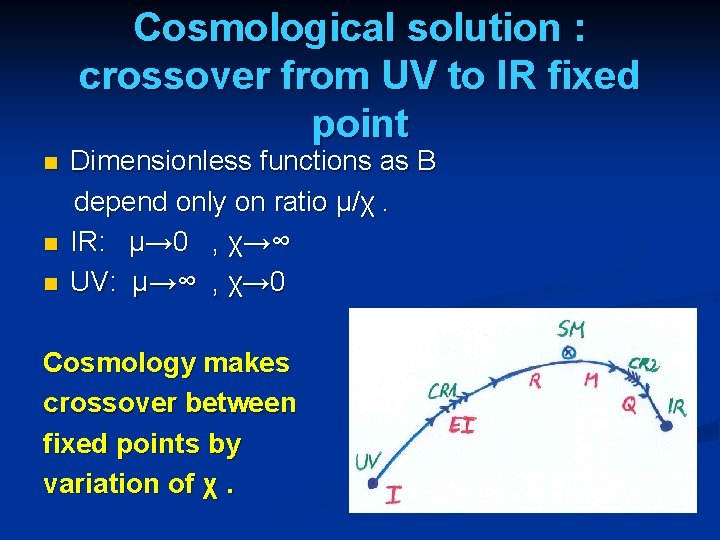Cosmological solution : crossover from UV to IR fixed point n n n Dimensionless functions as B depend only on ratio μ/χ. IR: μ→ 0 , χ→∞ UV: μ→∞ , χ→ 0 Cosmology makes crossover between fixed points by variation of χ.Simplicity simple description of all cosmological epochs natural incorporation of Dark Energy : n inflation n Early Dark Energy n present Dark Energy dominated epoch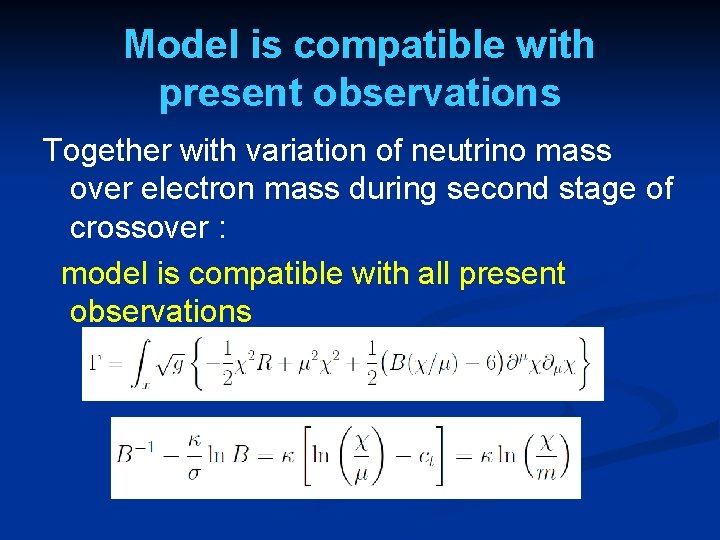Model is compatible with present observations Together with variation of neutrino mass over electron mass during second stage of crossover : model is compatible with all present observations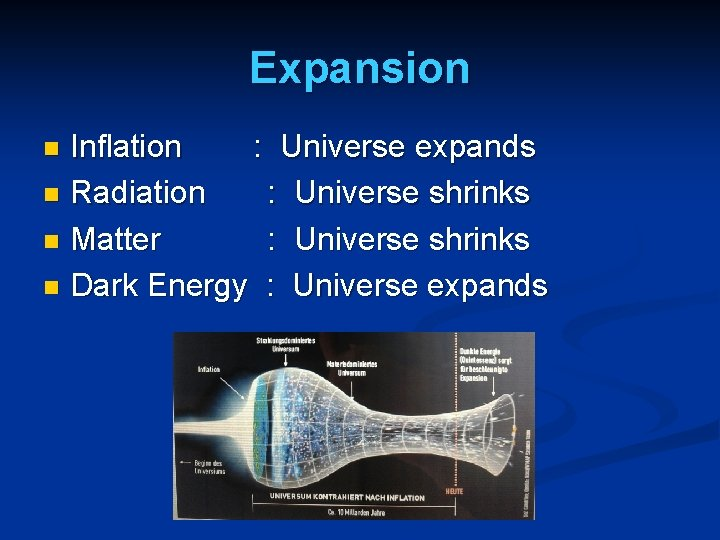Expansion Inflation : Universe expands n Radiation : Universe shrinks n Matter : Universe shrinks n Dark Energy : Universe expands n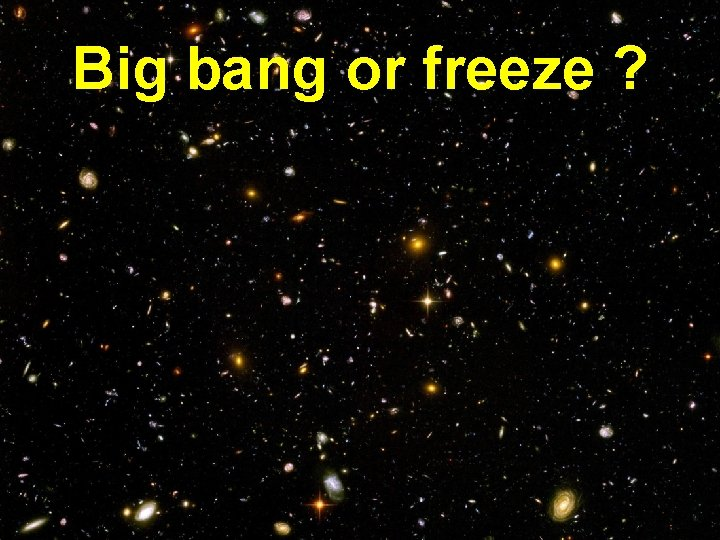Big bang or freeze ?NATURE | NEWS Cosmologist claims Universe may not be expanding Particles' changing masses could explain why distant galaxies appear to be rushing away. Jon Cartwright 16 July 2013 German physicist stops Universe 25. 07. 2013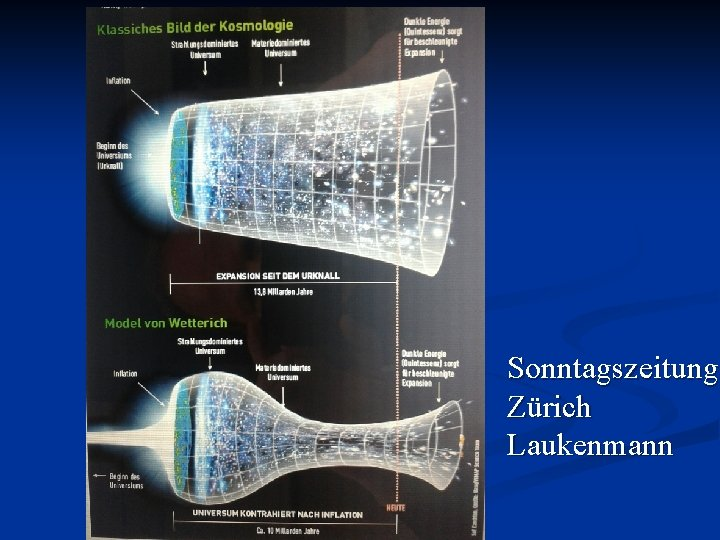Sonntagszeitung Zürich LaukenmannThe Universe is shrinkingThe Universe is shrinking … while Planck mass and particle masses are increasingRedshift instead of redshift due to expansion : smaller frequencies have been emitted in the past, because electron mass was smaller !What is increasing ? Ratio of distance between galaxies over size of atoms ! atom size constant : expanding geometry alternative : shrinking size of atoms general idea not new : Hoyle, Narlikar, …Different pictures of cosmology same physical content can be described by different pictures n related by field – redefinitions , e. g. Weyl scaling , conformal scaling of metric n which picture is usefull ? n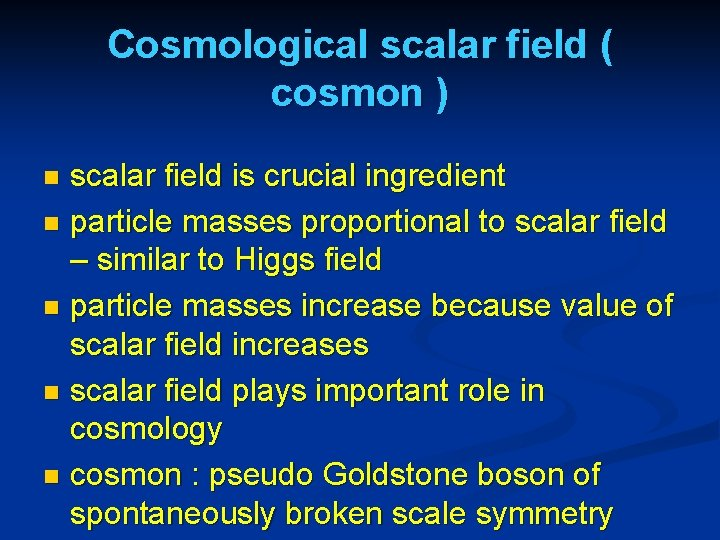Cosmological scalar field ( cosmon ) scalar field is crucial ingredient n particle masses proportional to scalar field – similar to Higgs field n particle masses increase because value of scalar field increases n scalar field plays important role in cosmology n cosmon : pseudo Goldstone boson of spontaneously broken scale symmetry nCosmon inflation Unified picture of inflation and dynamical dark energy Cosmon and inflaton are the same scalar fieldQuintessence Dynamical dark energy , generated by scalar field (cosmon ) C. Wetterich, Nucl. Phys. B 302(1988)668, 24. 9. 87 P. J. E. Peebles, B. Ratra, Ap. J. Lett. 325(1988)L 17, 20. 10. 87Prediction : homogeneous dark energy influences recent cosmology - of same order as dark matter Original models do not fit the present observations …. modifications ( different growth of neutrino mass )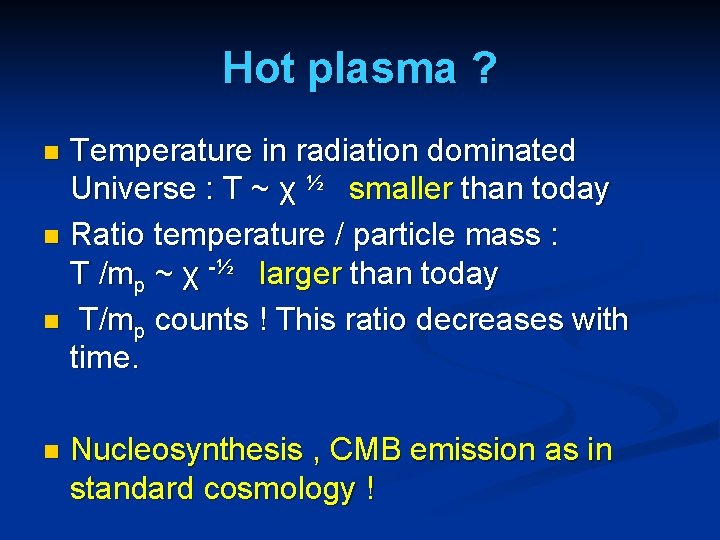Hot plasma ? Temperature in radiation dominated Universe : T ~ χ ½ smaller than today n Ratio temperature / particle mass : T /mp ~ χ -½ larger than today n T/mp counts ! This ratio decreases with time. n n Nucleosynthesis , CMB emission as in standard cosmology !Infinite past : slow inflation σ = 2 : field equations solutionSlow Universe μ= 2 10 -33 e. V Expansion or shrinking always slow , characteristic time scale of the order of the age of the Universe : tch ~ μ-1 ~ 10 billion years ! Hubble parameter of the order of present Hubble parameter for all times , including inflation and big bang ! Slow increase of particle masses !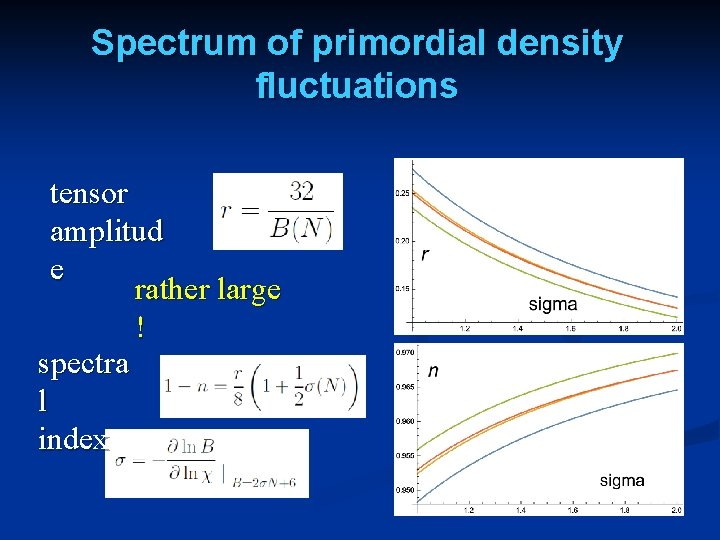Spectrum of primordial density fluctuations tensor amplitud e rather large ! spectra l indexAnomalous dimension determines spectrum of primordial fluctuations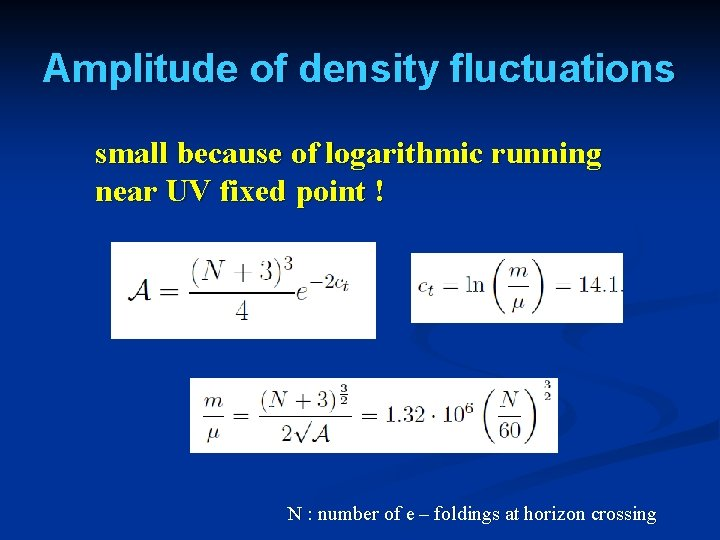Amplitude of density fluctuations small because of logarithmic running near UV fixed point ! N : number of e – foldings at horizon crossingFirst step of crossover ends inflation n induced by crossover in B n after crossover B changes only very slowlyScaling solutions near SM fixed point ( approximation for constant B ) Different scaling solutions for radiation domination and matter dominationconclusions n n n crossover in quantum gravity is reflected in crossover in cosmology quantum gravity becomes testable by cosmology quantum gravity plays a role not only for primordial cosmology crossover scenario explains different cosmological epochs simple model is compatible with present observations no more parameters than ΛCDM : tests possibleendRadiation domination Universe shrinks ! K=B-6 solution exists for B < 1 or K< 5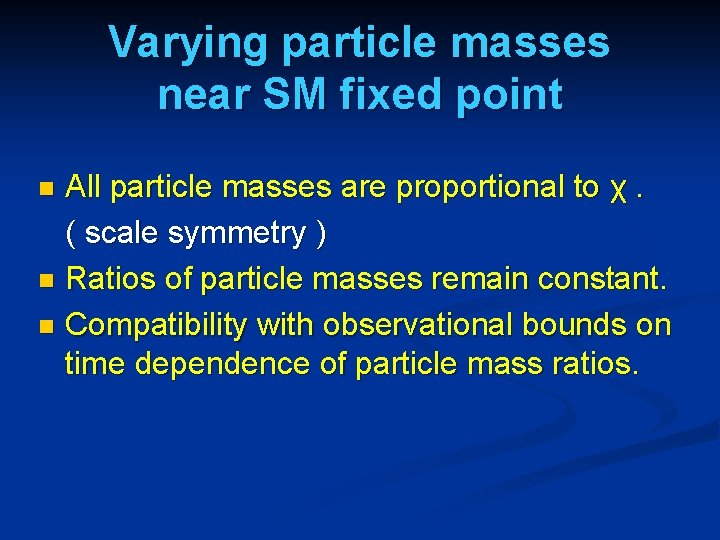Varying particle masses near SM fixed point All particle masses are proportional to χ. ( scale symmetry ) n Ratios of particle masses remain constant. n Compatibility with observational bounds on time dependence of particle mass ratios. n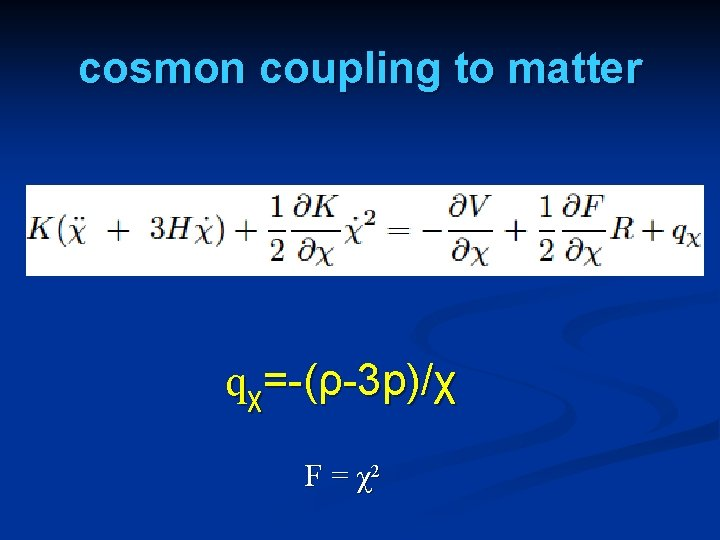cosmon coupling to matter qχ=-(ρ-3 p)/χ F = χ2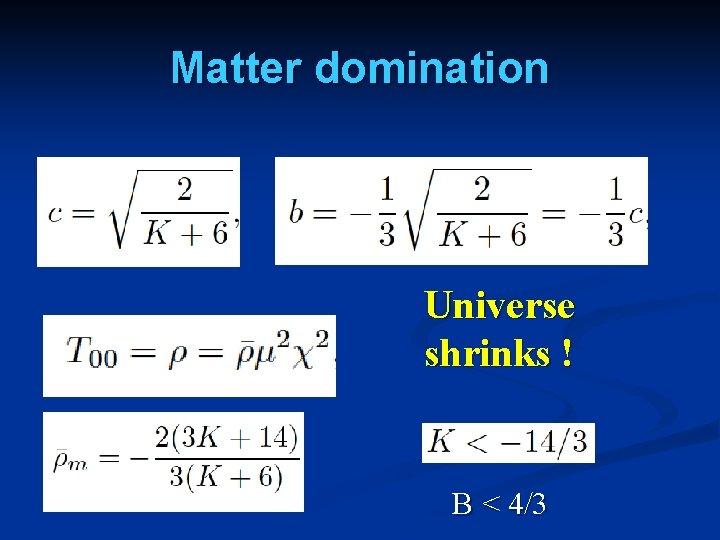Matter domination Universe shrinks ! B < 4/3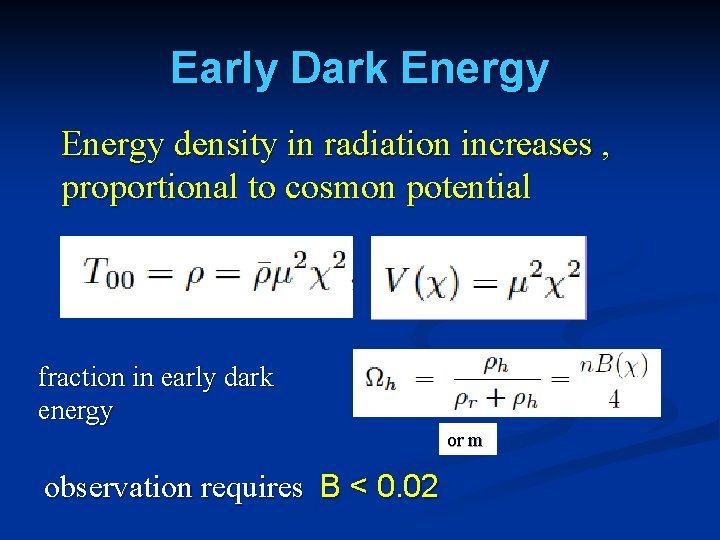Early Dark Energy density in radiation increases , proportional to cosmon potential fraction in early dark energy or m observation requires B < 0. 02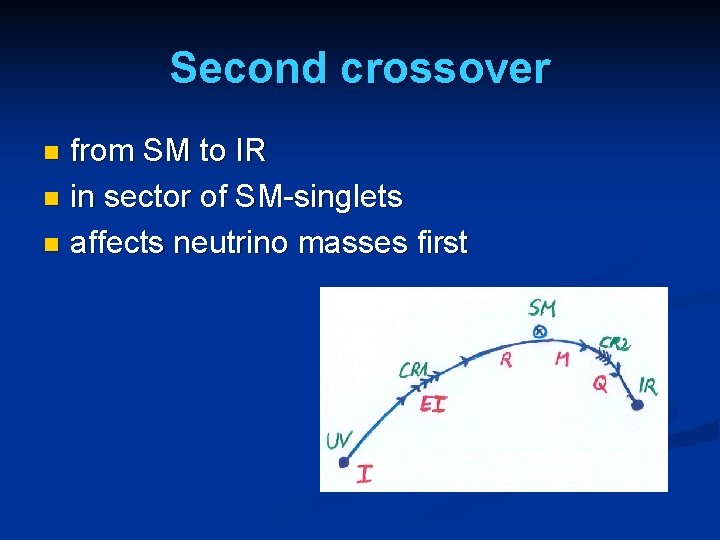Second crossover from SM to IR n in sector of SM-singlets n affects neutrino masses first n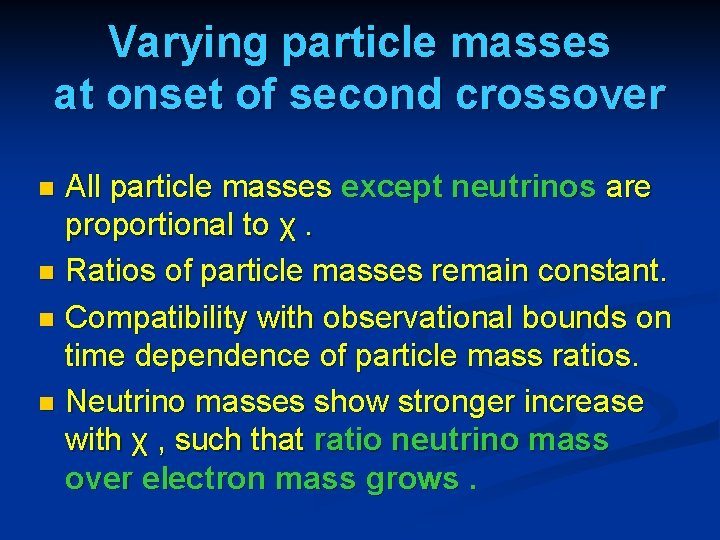Varying particle masses at onset of second crossover All particle masses except neutrinos are proportional to χ. n Ratios of particle masses remain constant. n Compatibility with observational bounds on time dependence of particle mass ratios. n Neutrino masses show stronger increase with χ , such that ratio neutrino mass over electron mass grows. n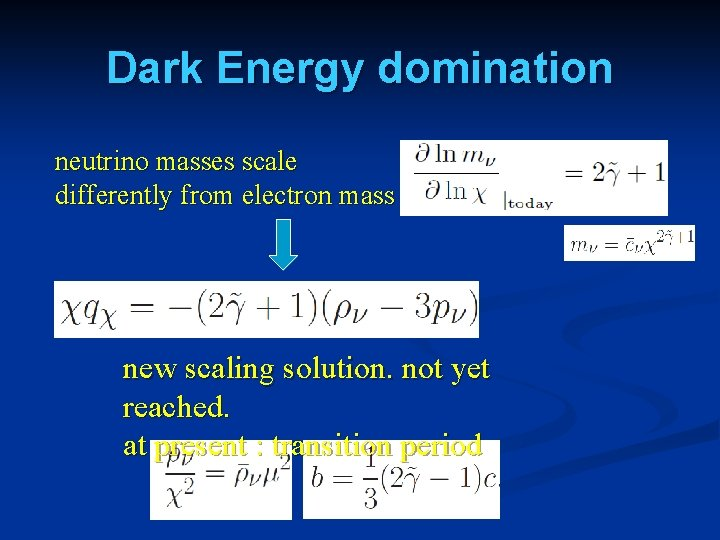Dark Energy domination neutrino masses scale differently from electron mass new scaling solution. not yet reached. at present : transition period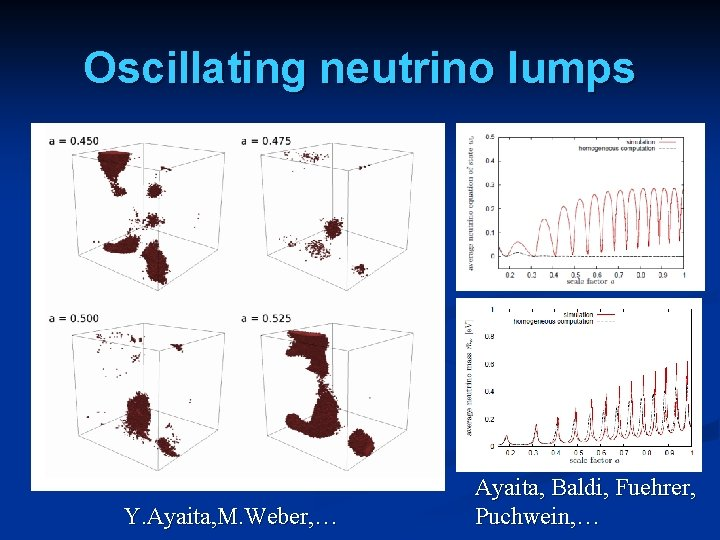Oscillating neutrino lumps Y. Ayaita, M. Weber, … Ayaita, Baldi, Fuehrer, Puchwein, …Evolution of dark energy similar to ΛCDMCompatibility with observations Realistic inflation model: n=0. 976 , r=0. 13 n Almost same prediction for radiation, matter, and Dark Energy domination as ΛCDM n Presence of small fraction of Early Dark Energy n Large neutrino lumps n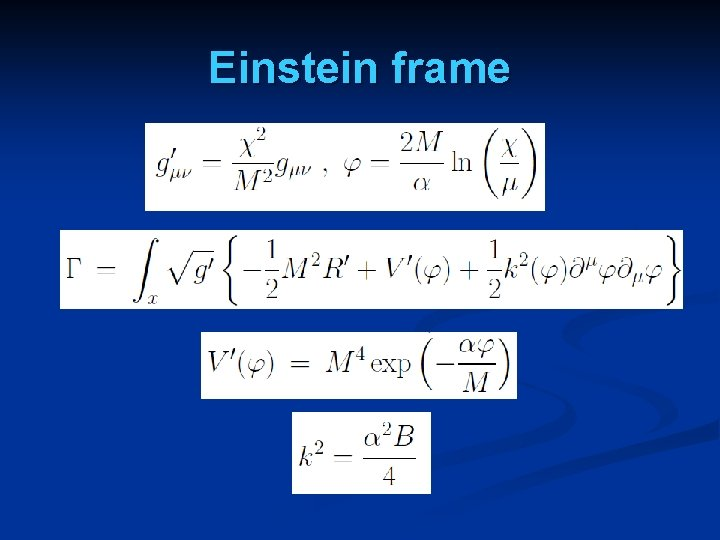Einstein frameEinstein frame n Weyl scaling maps variable gravity model to Universe with fixed masses and standard expansion history. n Standard gravity coupled to scalar field. n Only neutrino masses are growing.